NCERT Exemplar - MCQs

Chapter 6 Class 12 Application of Derivatives
Serial order wise

## (D) 1/2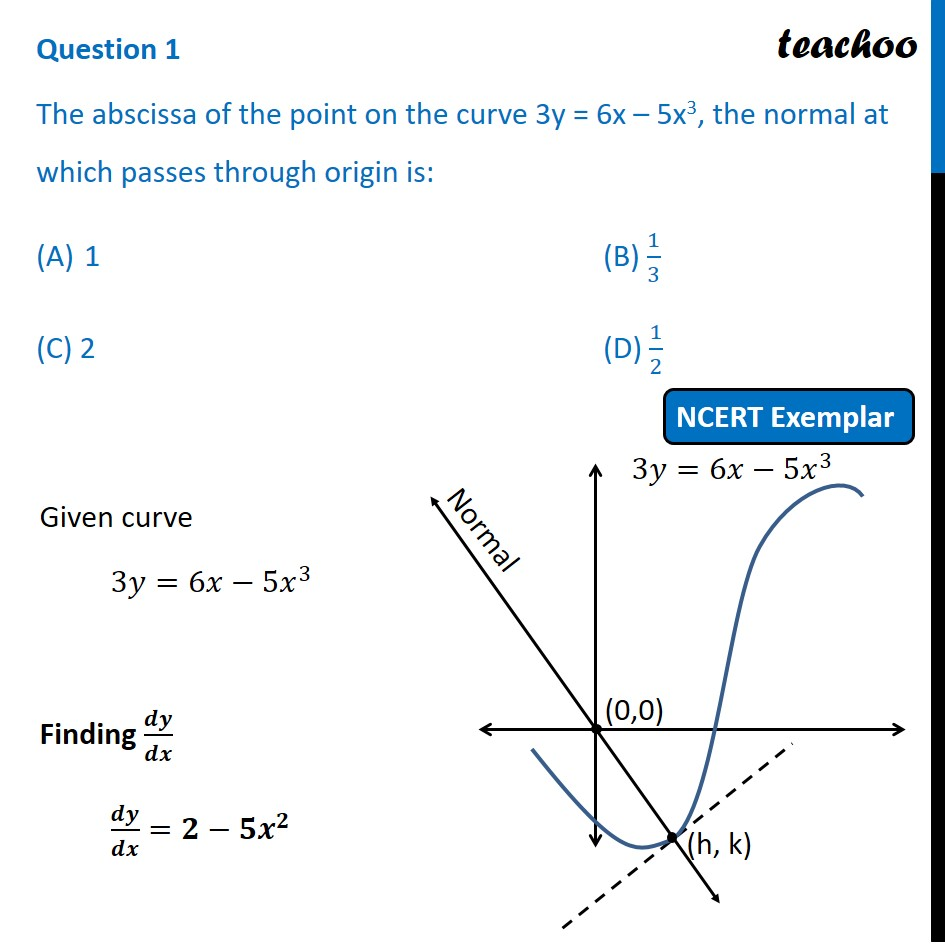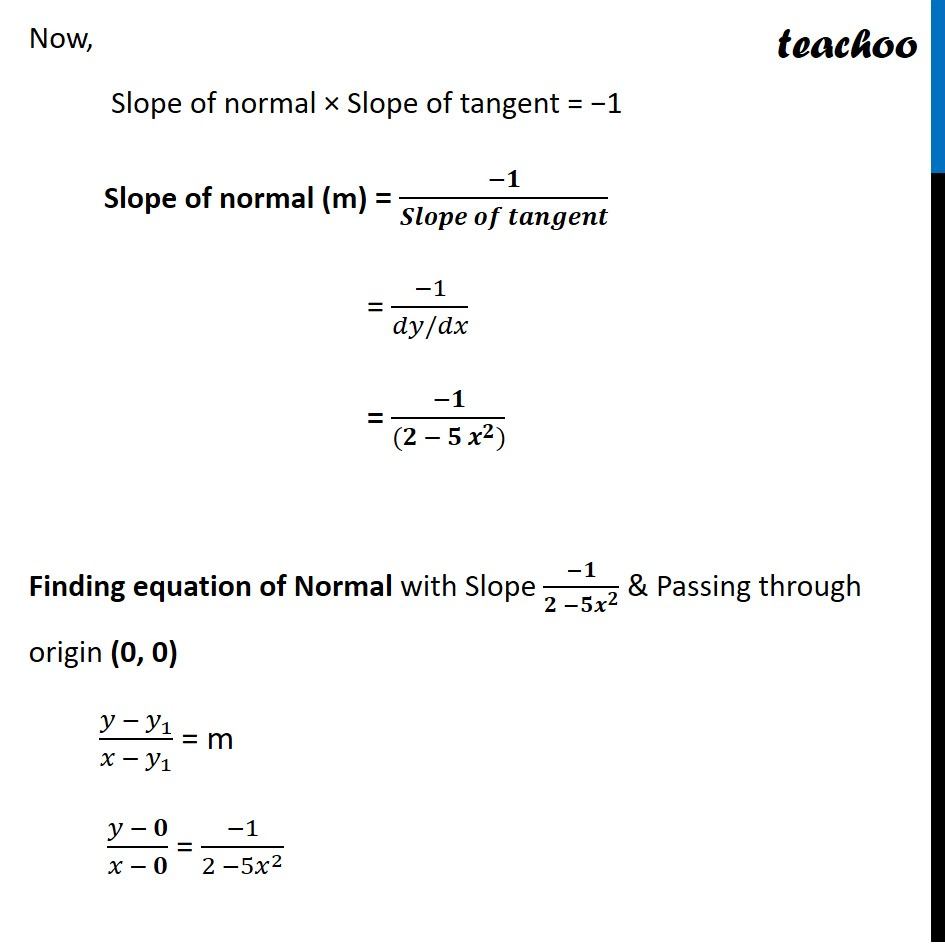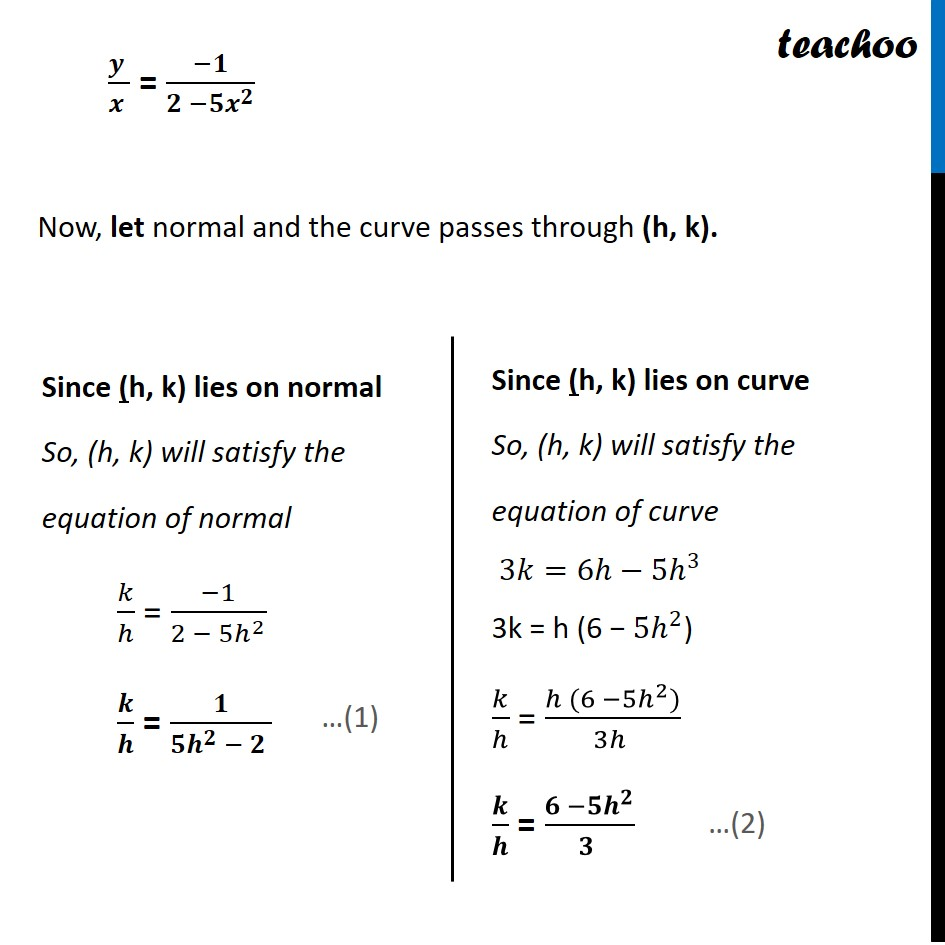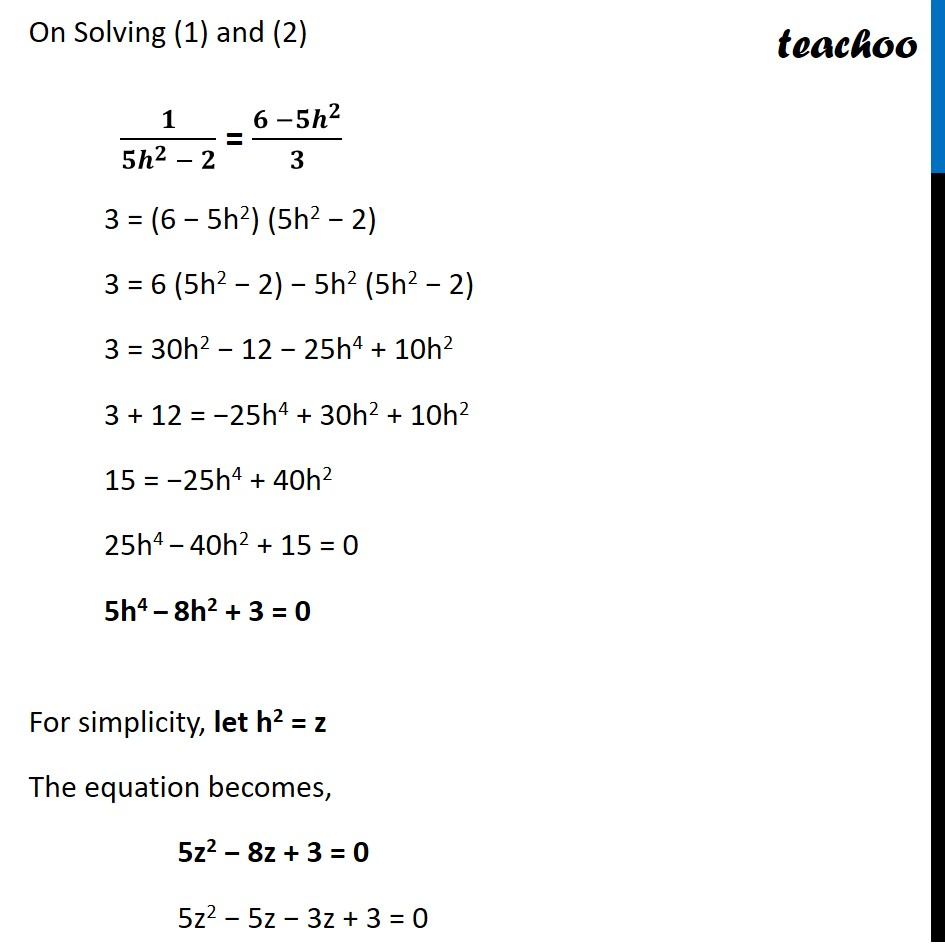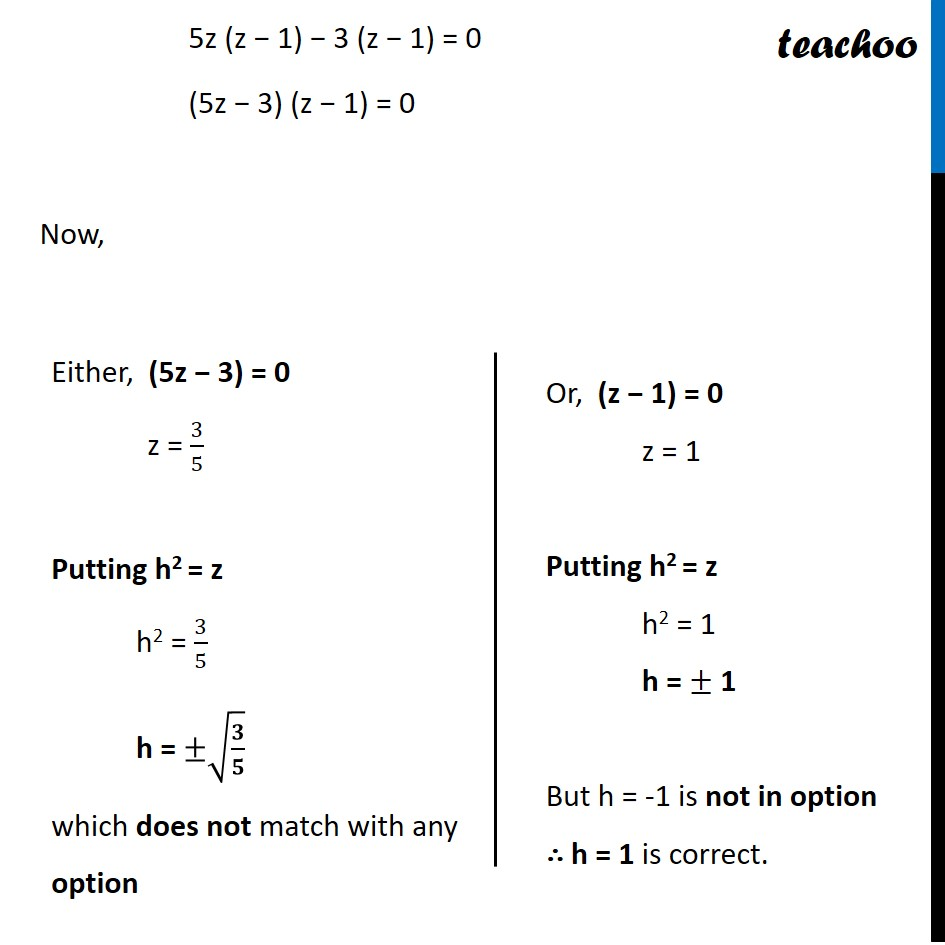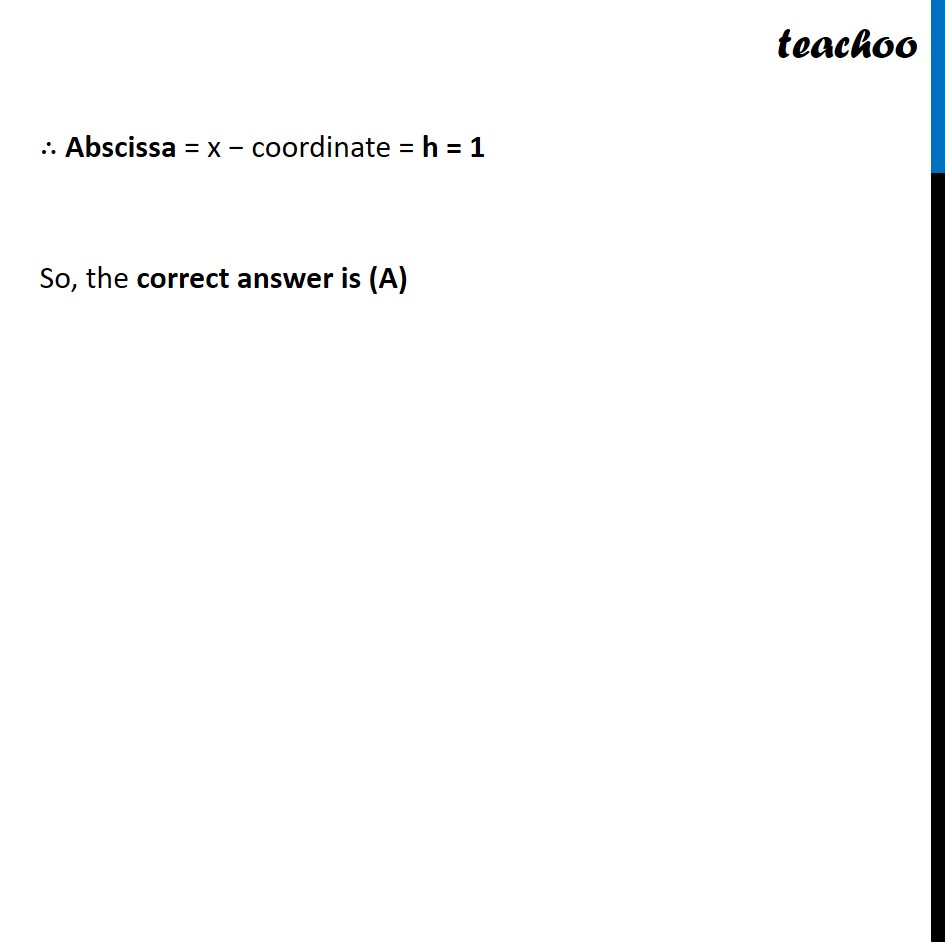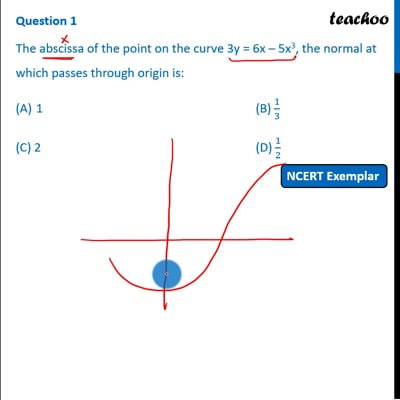This video is only available for Teachoo black users

Learn in your speed, with individual attention - Teachoo Maths 1-on-1 Class

### Transcript

Question 1 The abscissa of the point on the curve 3y = 6x – 5x3, the normal at which passes through origin is: 1 (B) 1/3 (C) 2 (D) 1/2 Given curve 3𝑦=6𝑥−5𝑥^3 Finding 𝒅𝒚/𝒅𝒙 𝒅𝒚/𝒅𝒙=𝟐−𝟓𝒙^𝟐 Now, Slope of normal × Slope of tangent = −1 Slope of normal (m) = (−𝟏)/(𝑺𝒍𝒐𝒑𝒆 𝒐𝒇 𝒕𝒂𝒏𝒈𝒆𝒏𝒕) = (−1)/(𝑑𝑦/𝑑𝑥) = (−𝟏)/((𝟐 − 𝟓 𝒙^𝟐)) Finding equation of Normal with Slope (−𝟏)/(𝟐 −𝟓𝒙^𝟐 ) & Passing through origin (0, 0) (𝑦 − 𝑦_1)/(𝑥 − 𝑦_1 ) = m (𝑦 − 𝟎)/(𝑥 − 𝟎) = (−1)/(2 −5𝑥^2 ) (𝒚 )/(𝒙 ) = (−𝟏)/(𝟐 −𝟓𝒙^𝟐 ) Now, let normal and the curve passes through (h, k). Since (h, k) lies on normal So, (h, k) will satisfy the equation of normal 𝑘/ℎ = (−1)/(2 − 5ℎ^2 ) 𝒌/𝒉 = 𝟏/(𝟓𝒉^𝟐 − 𝟐 ) Since (h, k) lies on curve So, (h, k) will satisfy the equation of curve 3𝑘=6ℎ−5ℎ^3 3k = h (6 − 5ℎ^2) 𝑘/ℎ = (ℎ (6 −5ℎ^2))/(3ℎ ) 𝒌/𝒉 = (𝟔 −𝟓𝒉^𝟐)/(𝟑 ) On Solving (1) and (2) 𝟏/(𝟓𝒉^𝟐 − 𝟐) = (𝟔 −𝟓𝒉^𝟐)/𝟑 3 = (6 − 5h2) (5h2 − 2) 3 = 6 (5h2 − 2) − 5h2 (5h2 − 2) 3 = 30h2 − 12 − 25h4 + 10h2 3 + 12 = −25h4 + 30h2 + 10h2 15 = −25h4 + 40h2 25h4 − 40h2 + 15 = 0 5h4 − 8h2 + 3 = 0 For simplicity, let h2 = z The equation becomes, 5z2 − 8z + 3 = 0 5z2 − 5z − 3z + 3 = 0 5z (z − 1) − 3 (z − 1) = 0 (5z − 3) (z − 1) = 0 Now, Either, (5z − 3) = 0 z = 3/5 Putting h2 = z h2 = 3/5 h = ±√(𝟑/𝟓) which does not match with any option Or, (z − 1) = 0 z = 1 Putting h2 = z h2 = 1 h = ± 1 But h = -1 is not in option ∴ h = 1 is correct. ∴ Abscissa = x − coordinate = h = 1 So, the correct answer is (A)